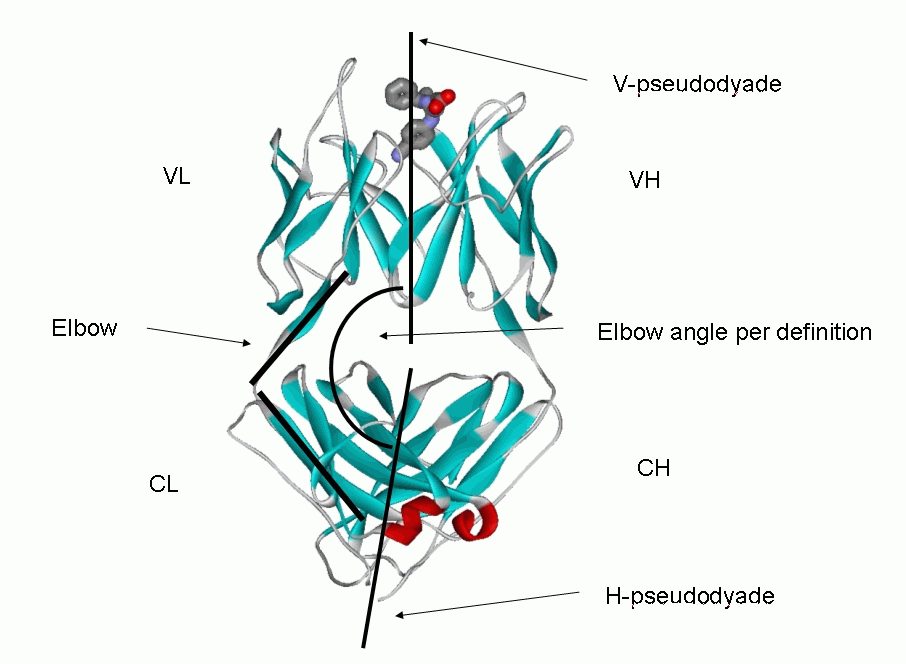Calculation of elbow angles

The elbow angle of a Fab antibody fragment is defined as the intersection angle of the two pseudo-dyad axes (PDAs) generated by the superposition of the light chain variable domain (VL) onto the heavy chain variable domain (VH), and of the light chain constant domain (CL) onto the heavy chain constant domain (CH1). As a measure for the relative orientation between the variable and the constant Fab domains, the elbow angle has been used in the comparison of liganded versus unliganded Fab structures and in assessment of Fab switch region flexibility.However, for a comparison between different Fabs on a common scale, two problems need to be addressed: First, the elbow angle calculated as the dot product of the VL-VH and CL-CH1 pseudo-dyad angle always computes between 0 and 180°. Although one could readily determine the absolute value in mathematical terms through the sign of the determinant of the basis matrix formed by the two pseudo-dyad vectors and their cross product vector, this does not overcome the problem: Due to the reduction of information to a single scalar angle value, the relative orientation of the axes is lost, and the solutions become degenerate (imaginable as located on a cone) between 90° to 180°.To regain the domain orientation on an absolute scale and to solve the complement ambiguity, one can compare each Fab to a ‘standard’ Fab with a defined elbow angle.  (we use the unliganded Fab 8F5 (PDB code = 1bbd) as a standard, elbow angle 127°). The Fab to be tested is first superimposed via its variable domain (VL-VH) onto the variable domain of the standard Fab. From this V-aligned orientation, the constant domain (CL-CH1) of the test Fab is aligned with the constant domain of the standard Fab, yielding the rotation relative to 1bbd. The sum of the standard Fab’s elbow angle (127°) plus the θ3 domain rotation angle is then used to resolve the degeneracy and to determine whether the elbow angle calculated by the dyad dot product is direct or complementary (> 180°). This method has been extensively used in previous reviews and compilations.